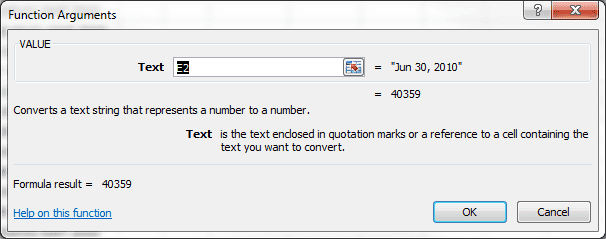# The VALUE Function in Excel – Converting Text Dates

The VALUE Function formula in cell G2 has converted the text string Date from cell E2, into a date serial number.

=VALUE(E2)

Until recently, I always used the VALUE Function to do this conversion. It’s something that’s well documented in the Function Arguments.

## Excel Value Function## Alternative Formulas to Convert Text Dates

However, all that’s required to change this text string Date into a numeric value (date serial number) is to perform a simple mathematical operation that does not change the value. Excel will then do the conversion for you.

The following formulas can be used as a substitute for the Excel value function =VALUE(E2), where E2 is the text string Date:

=E2 + 0

=E2 * 1

=E2 / 1

= – – E2

Each formula takes advantage of a simple mathematical operation: addition, multiplication, division, and double negation. Excel converts the text string Date into a Date serial number, in this case 40359, and then applies the mathematical operation(s). None of which will change the numeric value.

## Quick Conversion of Dates in a Range

To do a quick conversion on an entire range, John Walkenback, in his book Excel 2007 Formulas has a neat trick.

• Copy an empty cell
• Click the range with values
• Paste Special → In the dialog box select Add
• Then click OK

Excel converts text string Dates to serial numbers, then adds a zero and you end up with the date serial number. Pretty slick.

## Formatting a Date Serial Number

In all of these conversions you end up with a date serial number that can be changed to a Date by modifying the cell formatting. I use the keyboard shortcut Ctrl+1, select the Number tab and click Date.

In my next post I’ll use one formula to convert the text string date into a real numeric date.## 2 thoughts on “The VALUE Function in Excel – Converting Text Dates”

1.Horst Schmid

A user defined function like
Public Function DateFormatISO() As String
DateFormatISO = String(4, Application.International(xlYearCode)) & “-” _
& String(2, Application.International(xlMonthCode)) & “-” _
& String(2, Application.International(xlDayCode))
End Function
returns e.g. on a German system “JJJJ-MM-TT” and on a Englisch System “YYYY-MM-DD”. With that, the Worksheet function =TEXT(C4;DateFormatISO()) works independant from the users language and converts the serial date in cell C4 to an date string on any system!
Depending on whether Application.International(xlMDY) returns true or false, you may generate in a similar way a DateFormat string with month-day-year or day-month-year:
Public Function DateFormatString() As String
Dim s as string
s=Application.International(xlDateSeparator) ‘e.g. “/” or “.”
if(Application.International(xlMDY)) then
DateFormatString = String(2, Application.International(xlMonthCode)) & s _
& String(2, Application.International(xlDayCode)) & s _
& String(4, Application.International(xlYearCode))
else
DateFormatString = String(2, Application.International(xlDayCode)) & s _
& String(2, Application.International(xlMonthCode)) & s _
& String(4, Application.International(xlYearCode))
end if
End Function

1.Gregory

Thank you so much for sharing that code logic. 🙂 International date/time is tricky with VBA.